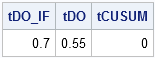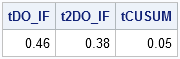Way back when I learned to program, I remember a computer instructor explaining that an IF-THEN statement can be a relatively slow operation. He said "If a multiplication takes one unit of time, an IF statement requires about 70 units." I don't know where his numbers came from, or even if they were correct, but I know that I spent a lot of time avoiding IF statements in order to make my programs run as fast as possible. I learned to write expressions such as y = (x>0) rather than the equivalent statement if (x>0) then y=1; else y=0.

Modern computers are much faster than they were back then, but old habits die hard. The following program was written by a colleague and examines the relative performances of a DO loop (=slow) versus a call to the CUSUM function (=fast) on a vector with one million elements. When I saw the program, I could hear my old instructor's voice whispering in my ear....watch out for those IF statements....

```proc iml; N = 1e6; z = rannorm(j(N,1)); /* generate random values */ y = j(N, 1, .);   /* time DO loop with compute cumulative sum (inefficient) */ t0 = time(); do i = 1 to n; if i = 1 then y[i] = z[i]; else y[i] = y[i-1] + z[i]; end; tDO_IF = time() - t0;   /* time vectorized computation (fast) */ t0 = time(); x = cusum(z); tCUSUM = time() - t0;```

As expected, the CUSUM function is much faster than writing a DO loop (the times are in the table that follows), which is why I always try to vectorize computations. That result was not surprising, but what caught my eye was the IF-THEN statement sitting inside of the DO loop. It occurred to me that part of the time spent by the first computation was because the IF-THEN statement is called one million times. To make a fairer comparison between the time taken by the DO loop and the CUSUM function, you can eliminate the IF-THEN statement entirely:

```t0 = time(); y=z; /* initialize */ do i = 2 to n; y[i] = y[i-1] + z[i]; end; tDO = time() - t0; print tDO_IF tDO tCUSUM;```The table shows that omitting the IF-THEN statement results in the DO loop running 20% faster.

## What if the IF-THEN statement is unavoidable?

You can't always eliminate an IF-THEN statement, but when you have an IF-THEN/ELSE statement inside a loop, you can usually restructure your program by writing two loops, one for when the IF condition is TRUE, and one for when it is FALSE. For example, consider the following statements that (inefficiently) compute two sums: the sum of the positive elements in a vector, and the sum of the negative elements:

```/* loop that "needs" an IF-THEN statement (?) */ /* IF-THEN inside loop...SLOW! */ t0 = time(); posSum = 0; negSum = 0; do i = 1 to n; if z[i] < 0 then negSum = negSum + z[i]; else posSum = posSum + z[i]; end; tDO_IF = time() - t0;   /* rewrite as two loops: one over positive elements and one over negative */ t0 = time(); negIdx = loc(z<0); posIdx = loc(z>0);; negSum = 0; do i = 1 to ncol(negIdx); negSum = negSum + z[negIdx[i]]; end; posSum = 0; do i = 1 to ncol(posIdx); posSum = posSum + z[posIdx[i]]; end; t2DO_IF = time() - t0;```

Of course, neither of these examples are as efficient as using a vectorized computation that calls the SUM function (see below). However, once again we observe a 20% improvement in the performance of the code by removing IF-THEN statements from inside the DO loop, as shown by the following table:

```/* vectorized computation (fast) */ t0 = time(); negIdx = loc(z<0); negSum = sum(z[negIdx]); posIdx = loc(z>0); posSum = sum(z[posIdx]); tCUSUM = time() - t0; print tDO_IF t2DO_IF tCUSUM;```Avoiding IF-THEN statements in a loop is just one of several ways to optimize loop performance. In general, you should remove any conditions that do not change inside of the loop. This is sometimes called "loop hoisting." For some compiled programming languages such as FORTRAN and C/C++, an optimizing compiler can sometimes determine that an expression is constant and move it outside of the loop for you.

So remember this little efficiency tip: avoid unnecessary statements inside loops. If it is easy to restructure the code to remove an IF-THEN statement inside a loop, or to move a constant expression outside of a loop, do so. It will pay off in terms of efficiency.

ShareDistinguished Researcher in Computational Statistics

Rick Wicklin, PhD, is a distinguished researcher in computational statistics at SAS and is a principal developer of SAS/IML software. His areas of expertise include computational statistics, simulation, statistical graphics, and modern methods in statistical data analysis. Rick is author of the books Statistical Programming with SAS/IML Software and Simulating Data with SAS.

1.What about the notion of trying to use as few lines of code as possible (ie, being succinct), rather than having two distinct do loops? Then, inside it you could have two statements:
negSum = negSum + (z[Idx[i]] < 0) * z[Idx[i]];
posSum = posSum + (z[Idx[i]] > 0) * z[Idx[i]];

2.I thought at first that I might be able to beat the vectorized solution above by using the 'choose' function, but I think it must be more or less equivalent to using 'loc'. Actually it is not necessary to construct two explicit (or implicit) indices of the elements of z, perhaps you would call this cheating, but I find that the following code runs around twice as fast.

t0 = time();
posSum = sum(choose(z>0,z,0));
negSum = posSum-sum(abs(z));
tCHOOSE = time() - t0;

So the cost for using loc or choose is large compared to running a simple function like abs() on every element of a matrix.

•For efficiency considerations of the CHOOSE function, see the article
"Complex assignment statements: CHOOSE wisely" and the associated comments.

•Your approach is a good one. Because the arguments of the CHOOSE function are so simple, CHOOSE is an efficient tool for this application.

3.Anders Sköllermo on

Hi! "If a multiplication takes one unit of time, an IF statement requires about 70 units."
Well, I would say that the opposite is true!.
Multiplications (especially of real numbers, which SAS always use) are usually expensive.
Logical comparisons are usually cheap.

BUT - everything is cheap compared to false solutions! Especially if they seem to be correct.
So, the earlier discussion by Rick about AND and SAS/IML is really great!

•There are definitely aspects here that are counterintuitive. The solution

negSum = sum(choose(z0,z,0));

runs in about two-thirds of the time that the two line solution based around the loc function takes, yet sum(choose(z<0,z,0)) must perform around twice as many additions as sum(z[loc(z<0)]). So additions at least can not be expensive. Any comments Rick?

•Additions are very cheap. Also, the solution with the LOC function requires more temporary arrays (memory allocations).

4.Richard Montes on

HI Rick,
I am simulating a r x c matrix. At each ith row, I have to calculate some statistic, say mean of the j columns. Depending on the value of the mean, I have to assign a value to a M vector using if-then statements. Is there a special way to define the M vector within an if-then loop? My code keeps giving me an error message "Matrix M has not been set to a value."
Thanks.
-Richard

•Post your (unworking) code to the SAS/IML Support Community.

•Richard Montes on

Rick,
I was able to make it work following your DO-loop in this example. I guess the key was defining the M vector, first with missing values. Then in the DO loop, assign M value at each ith row. Code works now. Thanks for all your examples in your blog. -Richard

====== working code ====
proc iml;

sim=j(5,10);
call randgen(sim, "Normal",100,0.5);

M = j(5,1,.);

sim_mean = sim[,:];

do i = 1 to 5;
if sim_mean[i] > 100 then M[i]=1;
else M[i]= 2;
end;

print M;

quit;

•Great! You could also eliminate the pre-allocation and the DO loop by using
M = choose(sim_mean > 100, 1, 2);
For an explanation, see my blog post on using the CHOOSE function.